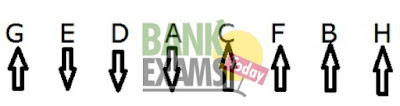# Reasoning Puzzles SBI Clerical 2016A, B, C, D, E, F, G and H are seated in a straight line but not necessarily in the same order Some of them are facing South while some are facing north .H sits fourth to left of A .A faces South. E sits
second to right of A. D sits second to right of G. G is neither an immediate neighbours of A nor H. Both the immediate neighbours of F face North. F is not an immediate neighbour of H .Immediate neighbours of E face opposite directions (i.e. if one neighbour faces north then the other faces south and vice versa). Both the immediate neighbours of B face the direction opposite to that of D (i.e. if D faces north then both the immediate neighbours of B face south and vice versa) E and C face opposite directions(i.e. if E faces north then C faces south and vice versa).

1.Which of the following pairs represents immediate neighbours of the persons seated at the  two extreme ends of the line ?

1).B, A
2).D, H
3).E, B
4).E, F
5).None

2. If each of the persons is made to sit in alphabetical order from right to left the positions of how many will remain unchanged as compared to the original seating arrangement ?

1).Three
2).Two
3).Four
4).One
5).None

3. What is the position of G with respect to A ?

1).Second to the left
2).Third to the right
3).Third to the left
4).Second to the right
5).Fifth to the right

4. How many persons are seated between A and B ?

1).Two
2).Four
3).Three
4).One
5).More than four

5. How many persons in the given arrangement are facing North ?

1).More than  Four
2).One
3).Three
4).Four
5).Two

SET 1 :1).3
2).4
3).2
4).1
5).1